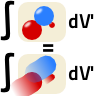## Lorentz Invariance of the Four-PotentialLorentz invariance is at the core of any (special) relativistic theory. Sometimes, however, we encounter expressions that seem to transform in a wrong way. This is especially true for the four-potential calculated from the retarded four-current. Find out why the four-potential is indeed Lorentz invariant!

## Problem Statement

The well-known retarded integrations over the charge density $$\rho\left(x^{\nu}\right)$$ and current density $$\mathbf{j}\left(x^{\nu}\right)$$ give rise to the scalar and vecor potential:$\begin{eqnarray*} \phi\left(x^{\nu}\right)&=&\frac{1}{4\pi\varepsilon_{0}}\int\frac{1}{\left|\mathbf{r}-\mathbf{r}^{\prime}\right|}\rho\left(\mathbf{r}^{\prime},t-\left|\mathbf{r}-\mathbf{r}^{\prime}\right|/c\right)dV^{\prime}\ \text{and}\\\mathbf{A}\left(x^{\nu}\right)&=&\frac{\mu_{0}}{4\pi}\int\frac{1}{\left|\mathbf{r}-\mathbf{r}^{\prime}\right|}\mathbf{j}\left(\mathbf{r}^{\prime},t-\left|\mathbf{r}-\mathbf{r}^{\prime}\right|/c\right)dV^{\prime}\ . \end{eqnarray*}$At a first glance, these equations seem to be not Lorentz invariant. Show that the four-potential $$A^{\mu}\left(x^{\nu}\right)=\left(\phi\left(x^{\nu}\right)/c,\,\mathbf{A}\left(x^{\nu}\right)\right)$$ obeys indeed Lorentz invariance:

• express $$A^{\mu}$$ in terms of the four-current $$j^{\mu}$$,
• re-forumlate the integral to go over space and time, $$dV^{\prime}\rightarrow dV^{\prime}dt^{\prime}$$ using the $$\delta$$-distribution and finally,
• $\text{use}\quad\delta\left[f\left(x\right)\right] = \sum_{\text{roots }x_{i}}\frac{\delta\left(x-x_{i}\right)}{\left|f^{\prime}\left(x_{i}\right)\right|}$ to find a forumulation of the integral without the $$1/\left|\mathbf{r}-\mathbf{r}^{\prime}\right|$$.